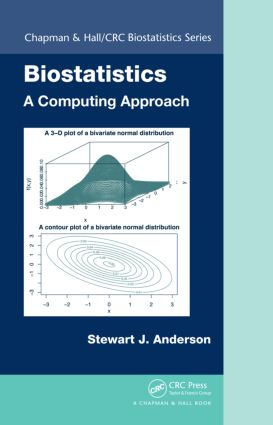Biostatistics: A Computing Approach

1st Edition

Chapman and Hall/CRC

328 pages | 65 B/W Illus.

Hardback: 9781584888345
pub: 2011-12-20
\$110.00
x

FREE Standard Shipping!

Description

The emergence of high-speed computing has facilitated the development of many exciting statistical and mathematical methods in the last 25 years, broadening the landscape of available tools in statistical investigations of complex data. Biostatistics: A Computing Approach focuses on visualization and computational approaches associated with both modern and classical techniques. Furthermore, it promotes computing as a tool for performing both analyses and simulations that can facilitate such understanding.

As a practical matter, programs in R and SAS are presented throughout the text. In addition to these programs, appendices describing the basic use of SAS and R are provided. Teaching by example, this book emphasizes the importance of simulation and numerical exploration in a modern-day statistical investigation. A few statistical methods that can be implemented with simple calculations are also worked into the text to build insight about how the methods really work.

Suitable for students who have an interest in the application of statistical methods but do not necessarily intend to become statisticians, this book has been developed from Introduction to Biostatistics II, which the author taught for more than a decade at the University of Pittsburgh.

Reviews

"The book presents important topics in biostatistics alongside examples provided in the programming languages SAS and R. … The book covers many relevant topics every student should know in a way that it makes it easy to follow … each chapter provides exercises encouraging the reader to deepen her/his understanding. I really like that the theory is presented in a clear manner without interruptions of example programs. Instead, the programs are always presented at the end of a section. … this book can serve as a good start for the more statistics inclined students who haven’t yet recognized that in order to become a good biostatistician, you need to be able to write your own code. … I can recommend to all serious students who want to get a thorough start into this field."

—Frank Emmert-Streib, Queen’s University Belfast, CHANCE, August 2013

Preface

Review of Topics in Probability and Statistics

Introduction to Probability

Conditional Probability

Random Variables

The Uniform distribution

The Normal distribution

The Binomial Distribution

The Poisson Distribution

The Chi–Squared Distribution

Student’s t–distribution

The F-distribution

The Hypergeometric Distribution

The Exponential Distribution

Exercises

Use of Simulation Techniques

Introduction

What can we accomplish with simulations?

How to employ a simple simulation strategy

Generation of Pseudorandom Numbers

Generating Discrete and Continuous random variables

Testing Random Number Generators

A Brief Note on the Efficiency of Simulation Algorithms

Exercises

The Central Limit Theorem

Introduction

The Strong Law of Large Numbers

The Central Limit Theorem

Summary of the Inferential Properties of the Sample Mean

Appendix: Program Listings

Exercises

Correlation and Regression

Introduction

Pearson’s Correlation Coefficient

Simple Linear Regression

Multiple Regression

Visualization of Data

Model Assessment and Related Topics

Polynomial Regression

Smoothing Techniques

Appendix: A Short Tutorial in Matrix Algebra

Exercises

Analysis of Variance

Introduction

One–Way Analysis of Variance

General Contrast

Multiple Comparisons Procedures

Gabriel’s method

Dunnett’s Procedure

Two-Way Analysis of Variance: Factorial Design

Two-Way Analysis of Variance: Randomized Complete Blocks

Analysis of Covariance

Exercises

DiscreteMeasures of Risk

Introduction

Odds Ratio (OR) and Relative Risk (RR)

Calculating risk in the presence of confounding

Logistic Regression

Using SAS and R for Logistic Regression

Comparison of Proportions for Paired Data

Exercises

Multivariate Analysis

The Multivariate Normal Distribution

One and Two Sample Multivariate Inference

Multivariate Analysis of Variance

Multivariate Regression Analysis

Classification Methods

Exercises

Analysis of Repeated Measures Data

Introduction

Plotting Repeated Measures Data

Univariate Approaches for the Analysis of Repeated Measures Data

Covariance Pattern Models

Multivariate Approaches

Modern Approaches for the Analysis of Repeated Measures Data

Analysis of Incomplete Repeated Measures Data

Exercises

NonparametricMethods

Introduction

Comparing Paired Distributions

Comparing Two Independent Distributions

Kruskal–Wallis Test

Spearman’s rho

The Bootstrap

Exercises

Analysis of Time to Event Data

Incidence Density (ID)

Introduction to Survival Analysis

Estimation of the Survival Curve

Estimating the Hazard Function

Comparing Survival in Two Groups

Cox Proportional Hazards Model

Cumulative Incidence

Exercises

Sample size and power calculations

Sample sizes and power for tests of normally distributed data

Sample size and power for Repeated Measures Data

Sample size and power for survival analysis

Constructing Power Curves

Exercises

Appendix A: Using SAS

Introduction

Data input in SAS

Some Graphical Procdures: PROC PLOT and PROC CHART

Some Simple Data Analysis Procedures

Diagnosing errors in SAS programs

Exercises

Appendix B: Using R

Introduction

Getting started

Input/Output

Some Simple Data Analysis Procedures

Using R for plots

Comparing an R–session to a SAS session

Diagnosing problems in R programs

Exercises

References

Index